calc.thetimetube.com
•  70,844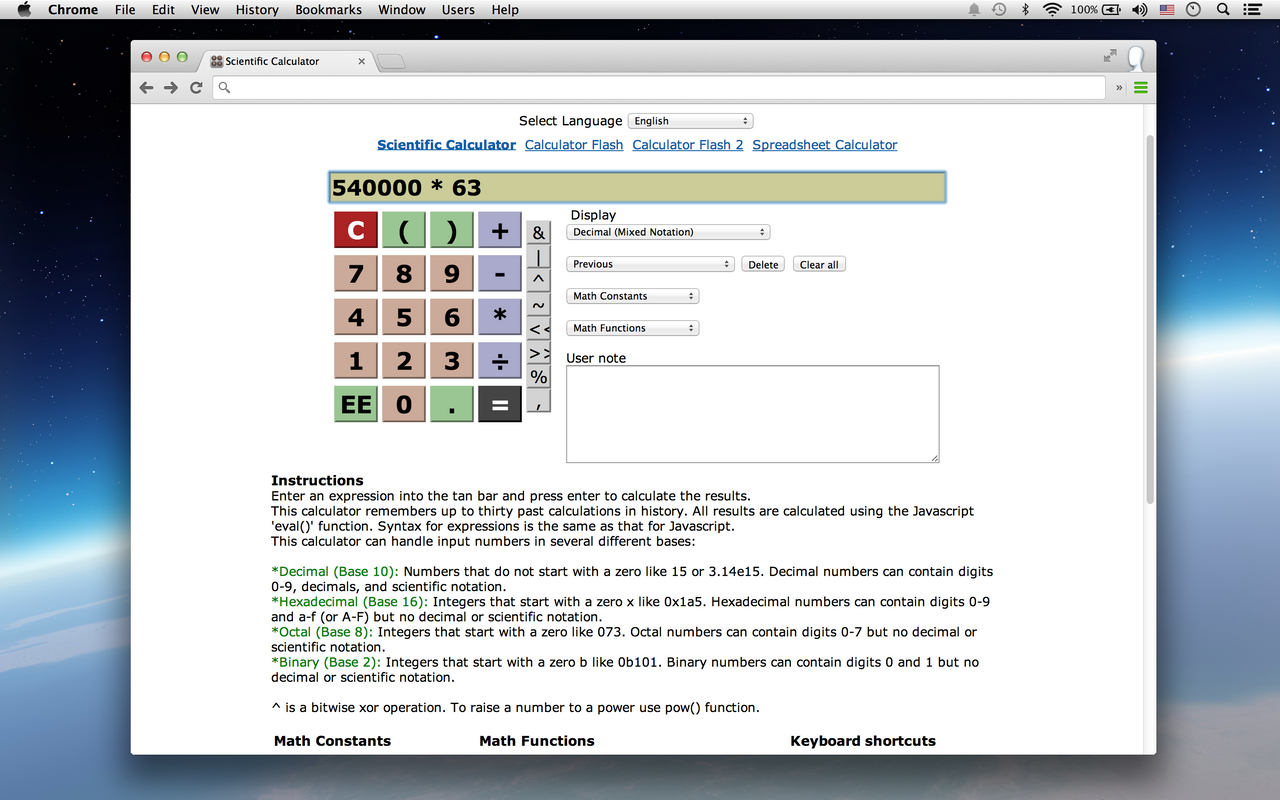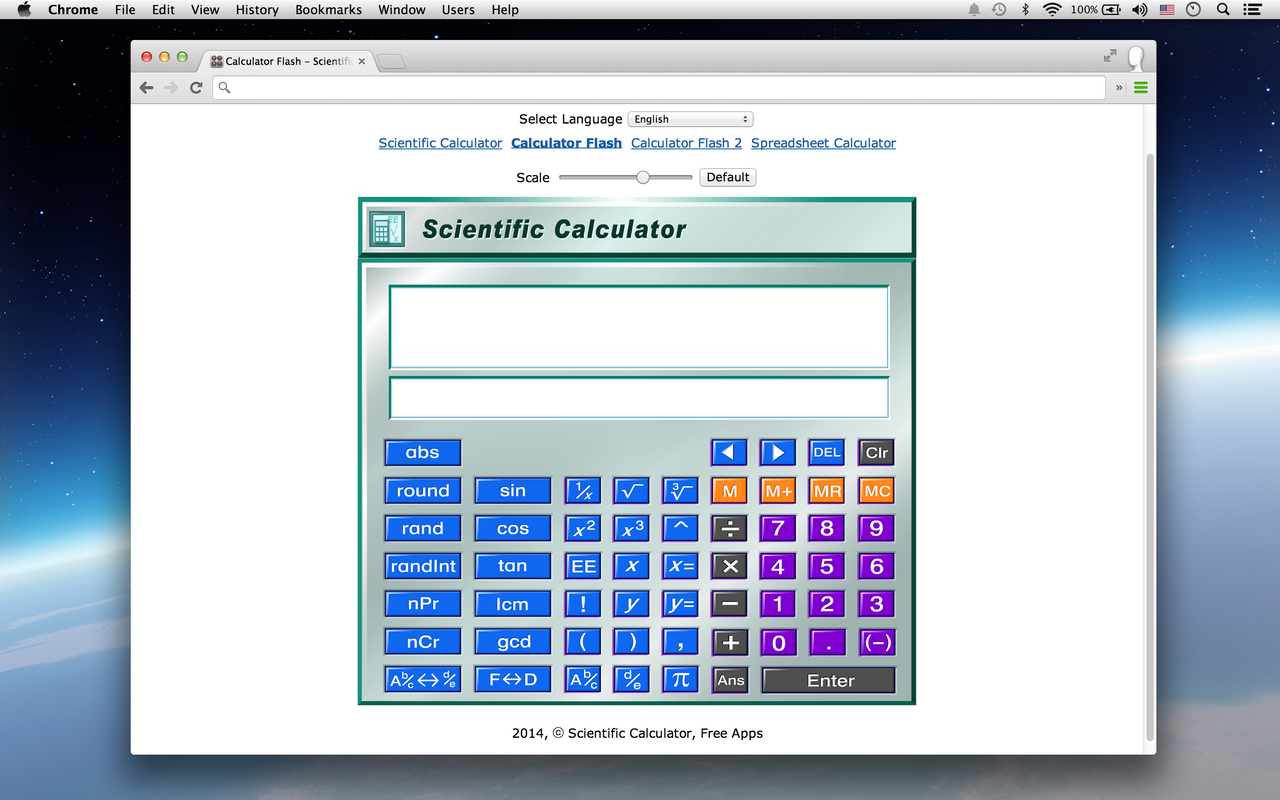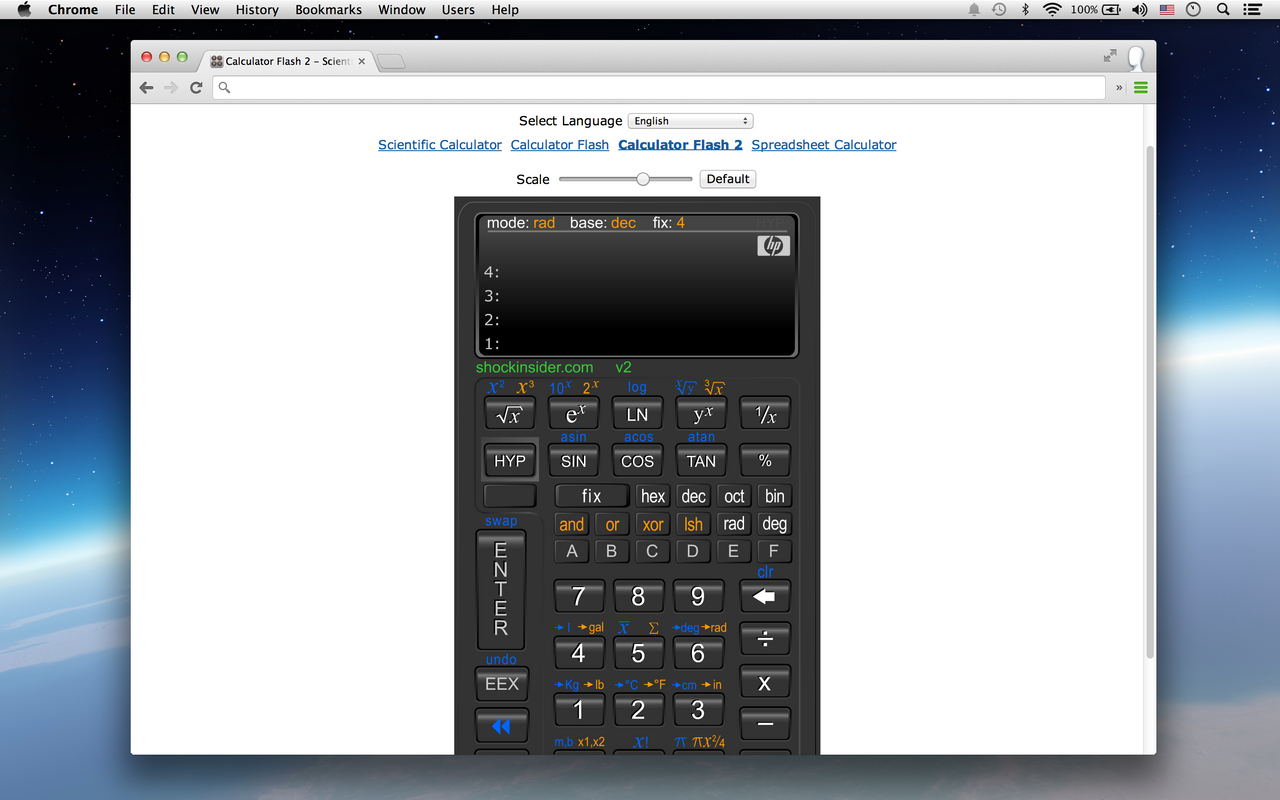Overview
Free calculator, unit converter for general, students, Mathematics, Engineering. Easy-to-use General or Scientific Calculator.
```This app is a free general,math/scientific calculator similar to those from TI, Casio, HP and others. It supports functions for algebra and trigonometry. Enter a formula either by typing on the keyboard, pressing the buttons on the calculator with the mouse, or a mixture of both.

Support Math Constants, Math Functions, Keyboard shortcuts...

Free calculator for general, students, advanced Mathematics, Physics and Engineering problems.
Easy-to-use General Purpose Calculator, Scientific Calculator.

Provides Total Unit Converter.
It is easily convert various units to different measurement systems. Entry the required value, it will get the results automatically.```
Reviews
Language:
Sort by: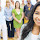Samson Assefa
December 13, 2020
Samson Assefa Gebrehiwot
Yes
No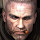A User of Scientific Calculator, Unit Converter
May 6, 2018
This is not a caculator app. ITS THE FUTURE OF COMPUTERS. You only need a connection. They will do the rest so you dont need to.
Yes
No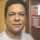A User of Scientific Calculator, Unit Converter
May 30, 2017
Very helpful to organize handy data......
Yes
No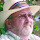A User of Scientific Calculator, Unit Converter
April 9, 2017
Useless. No log10
Yes
No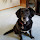A User of Scientific Calculator, Unit Converter
August 12, 2016
Lasted about 30 seconds before deleting.Basicly just a unit converter.
Yes
NoA User of Scientific Calculator, Unit Converter
March 1, 2015
so good
Yes
No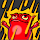A User of Scientific Calculator, Unit Converter
November 13, 2013
"Easy To Use" No, I couldn't figure this out at all. Doubt it could solve equations anyway...
Yes
No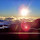A User of Scientific Calculator, Unit Converter
September 28, 2013
not like normal scientific calculator
Yes
NoA User of Scientific Calculator, Unit Converter
September 21, 2013
I thought it would solve equations for you. It does not even let you have fractions. I advise you not to even download this app. It is a waste of time. You cannot even substitute fractions with a slanted line. Sorry I do not know what is is called